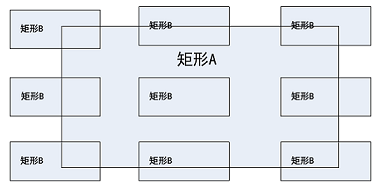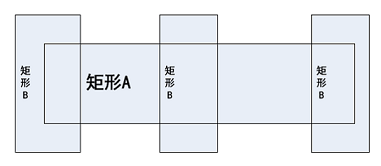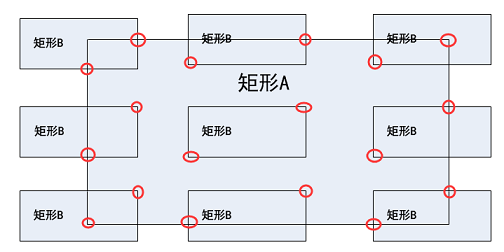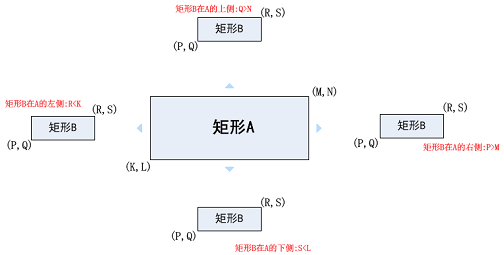• 两个矩形边角相交以及完全包含• 矩形横向相交• 矩形纵向相交• 矩形被完全包含``````1 2 3 4 5 6 7 8 `````` ``````public static int calculateIntersectArea(int k, int l, int m, int n, int p,int q, int r, int s) { int leftDownX = 0, leftDownY = 0, rightUpX = 0, rightUpY = 0; leftDownX = k > p ? k : p; leftDownY = l > q ? l : q; rightUpX = m < r ? m : r; rightUpY = n < s ? n : s; return (rightUpX - leftDownX) * (rightUpY - leftDownY); } ```````````` 1 2 3 4 5 6 7 8 9 10 11 12 13 14 `````` ``````public static boolean isIntersect(int k, int l, int m, int n, int p, int q, int r, int s) { boolean nointersect = false; // B在A的上面 nointersect = q > n; // B在A的下面 nointersect = l > s; // B在A的左侧 nointersect = r < k; // B在A的右侧 nointersect = m < p; return !nointersect; } ``````

 `````` 1 2 3 4 5 6 7 8 9 10 11 12 13 14 15 16 17 `````` ``````public static int solution(int k, int l, int m, int n, int p, int q, int r, int s) { int widthA = m - k; int heightA = n - l; int areaA = widthA * heightA; int widthB = r - p; int heightB = s - q; int areaB = widthB * heightB; int totalArea = areaA + areaB; boolean hasIntersect = isIntersect(k, l, m, n, p, q, r, s); if (hasIntersect) { totalArea -= calculateIntersectArea(k, l, m, n, p, q, r, s); } return totalArea; } ``````